# HSSlive: Plus One & Plus Two Notes & Solutions for Kerala State Board

## AP Board Class 7 Maths Chapter 14 Understanding 3D and 2D Shapes Ex 3 Textbook Solutions PDF: Download Andhra Pradesh Board STD 7th Maths Chapter 14 Understanding 3D and 2D Shapes Ex 3 Book AnswersAP Board Class 7 Maths Chapter 14 Understanding 3D and 2D Shapes Ex 3 Textbook Solutions PDF: Download Andhra Pradesh Board STD 7th Maths Chapter 14 Understanding 3D and 2D Shapes Ex 3 Book Answers

## Andhra Pradesh State Board Class 7th Maths Chapter 14 Understanding 3D and 2D Shapes Ex 3 Books Solutions

 Board AP Board Materials Textbook Solutions/Guide Format DOC/PDF Class 7th Subject Maths Chapters Maths Chapter 14 Understanding 3D and 2D Shapes Ex 3 Provider Hsslive

## How to download Andhra Pradesh Board Class 7th Maths Chapter 14 Understanding 3D and 2D Shapes Ex 3 Textbook Solutions Answers PDF Online?

2. Click on the Andhra Pradesh Board Class 7th Maths Chapter 14 Understanding 3D and 2D Shapes Ex 3 Answers.
3. Look for your Andhra Pradesh Board STD 7th Maths Chapter 14 Understanding 3D and 2D Shapes Ex 3 Textbooks PDF.
4. Now download or read the Andhra Pradesh Board Class 7th Maths Chapter 14 Understanding 3D and 2D Shapes Ex 3 Textbook Solutions for PDF Free.

## AP Board Class 7th Maths Chapter 14 Understanding 3D and 2D Shapes Ex 3 Textbooks Solutions with Answer PDF Download

Find below the list of all AP Board Class 7th Maths Chapter 14 Understanding 3D and 2D Shapes Ex 3 Textbook Solutions for PDF’s for you to download and prepare for the upcoming exams:

Question 1.
Use an isometric dot paper and make an isometric sketch for each one of the given shapes.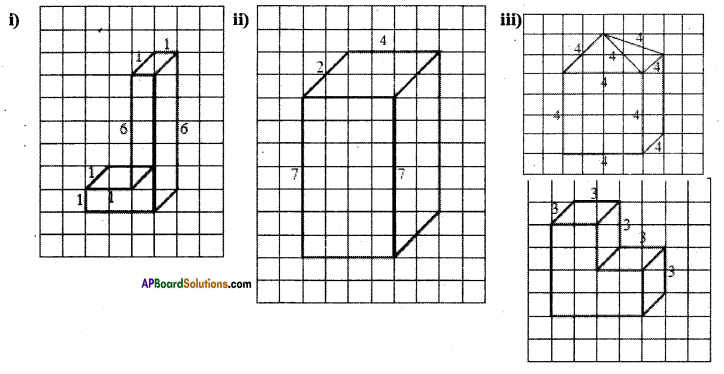Solution: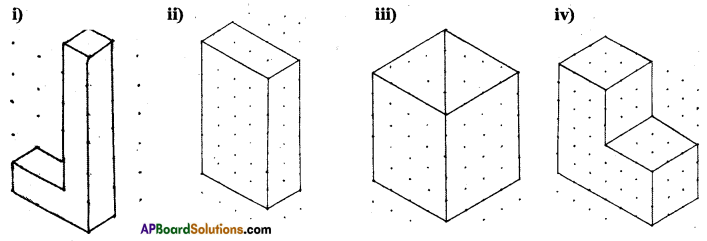Question 2.
The dimensions of a cuboid are 5 cm, 3 cm and 2 cm. Draw three different isometric sketches of this cuboid.
Solution: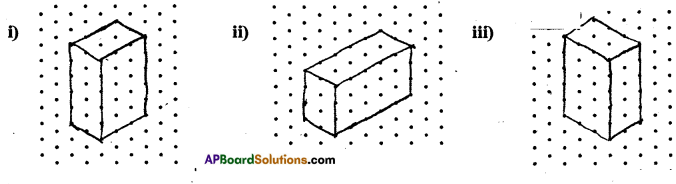Question 3.
Three cubes each with 2 cm edge are placed side by side to form a cuboid. Draw an oblique or isometric sketch of this cuboid.
Solution: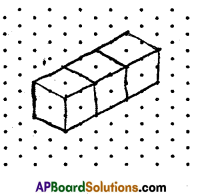Question 4.
Make an oblique sketch for each of the given isometric shapes.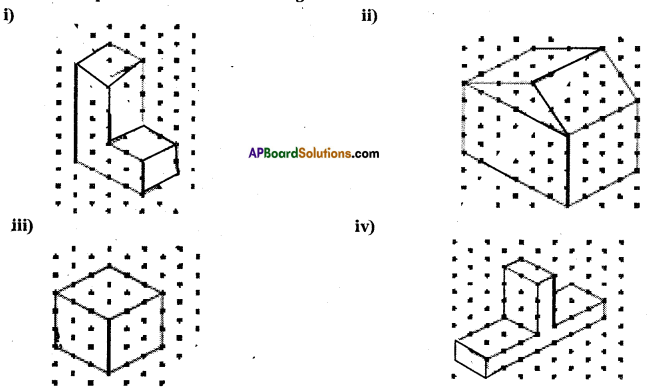Solution: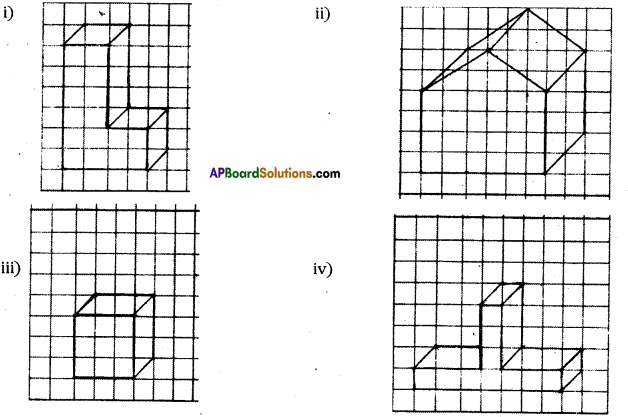Question 5.
Give (i) an oblique sketch and (ii) an isometric sketch for each of the following:
(a) A cuboid of dimensions 5 cm, 3 cm and 2 cm. (Is your sketch unique’?)
(b) A cube with an edge 4 cm long.
Solution: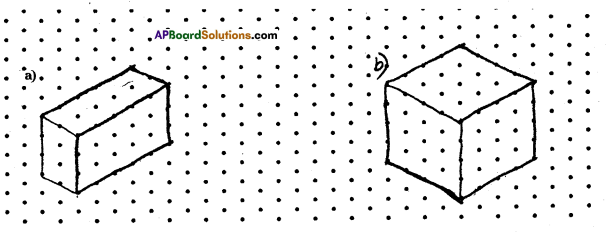## Andhra Pradesh Board Class 7th Maths Chapter 14 Understanding 3D and 2D Shapes Ex 3 Textbooks for Exam Preparations

Andhra Pradesh Board Class 7th Maths Chapter 14 Understanding 3D and 2D Shapes Ex 3 Textbook Solutions can be of great help in your Andhra Pradesh Board Class 7th Maths Chapter 14 Understanding 3D and 2D Shapes Ex 3 exam preparation. The AP Board STD 7th Maths Chapter 14 Understanding 3D and 2D Shapes Ex 3 Textbooks study material, used with the English medium textbooks, can help you complete the entire Class 7th Maths Chapter 14 Understanding 3D and 2D Shapes Ex 3 Books State Board syllabus with maximum efficiency.

## FAQs Regarding Andhra Pradesh Board Class 7th Maths Chapter 14 Understanding 3D and 2D Shapes Ex 3 Textbook Solutions

#### Can we get a Andhra Pradesh State Board Book PDF for all Classes?

Yes you can get Andhra Pradesh Board Text Book PDF for all classes using the links provided in the above article.

## Important Terms

Andhra Pradesh Board Class 7th Maths Chapter 14 Understanding 3D and 2D Shapes Ex 3, AP Board Class 7th Maths Chapter 14 Understanding 3D and 2D Shapes Ex 3 Textbooks, Andhra Pradesh State Board Class 7th Maths Chapter 14 Understanding 3D and 2D Shapes Ex 3, Andhra Pradesh State Board Class 7th Maths Chapter 14 Understanding 3D and 2D Shapes Ex 3 Textbook solutions, AP Board Class 7th Maths Chapter 14 Understanding 3D and 2D Shapes Ex 3 Textbooks Solutions, Andhra Pradesh Board STD 7th Maths Chapter 14 Understanding 3D and 2D Shapes Ex 3, AP Board STD 7th Maths Chapter 14 Understanding 3D and 2D Shapes Ex 3 Textbooks, Andhra Pradesh State Board STD 7th Maths Chapter 14 Understanding 3D and 2D Shapes Ex 3, Andhra Pradesh State Board STD 7th Maths Chapter 14 Understanding 3D and 2D Shapes Ex 3 Textbook solutions, AP Board STD 7th Maths Chapter 14 Understanding 3D and 2D Shapes Ex 3 Textbooks Solutions,
Share: